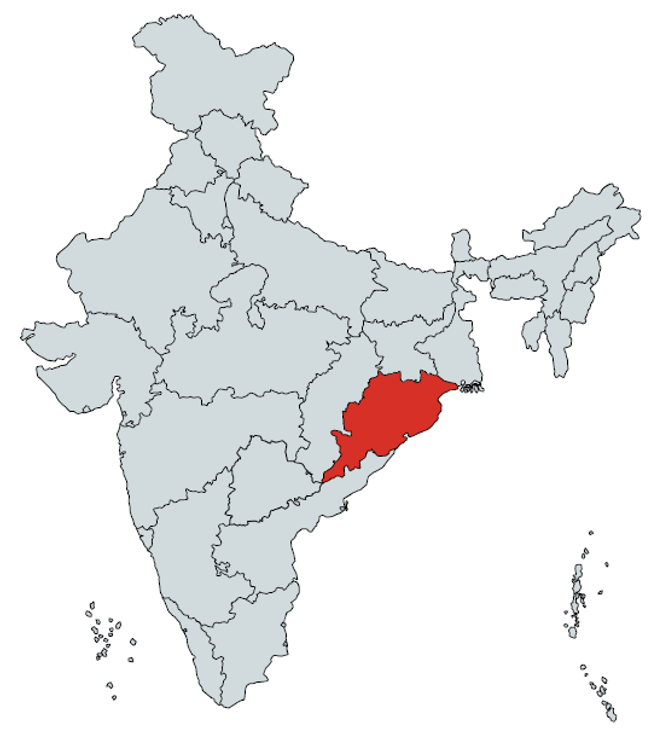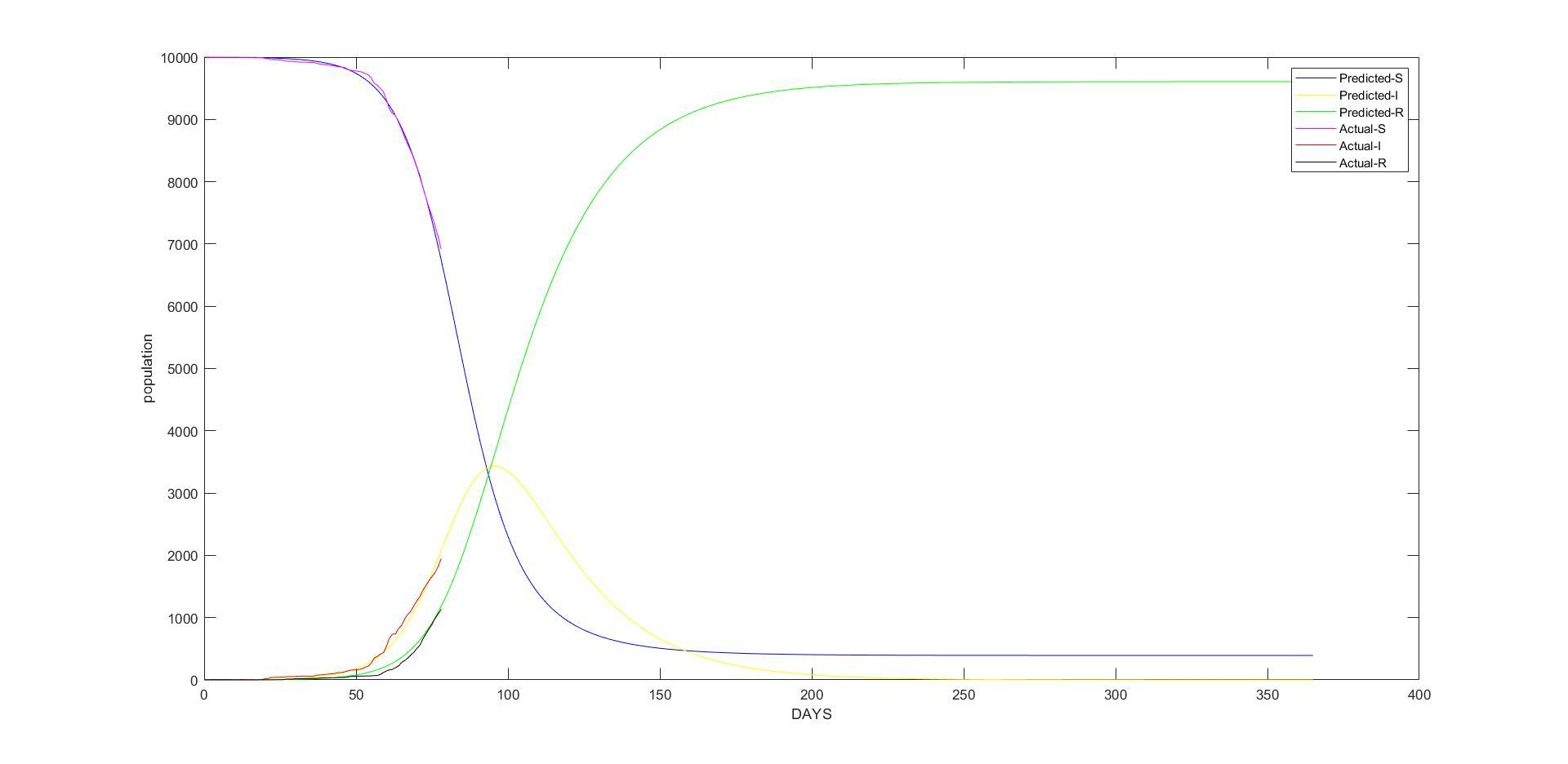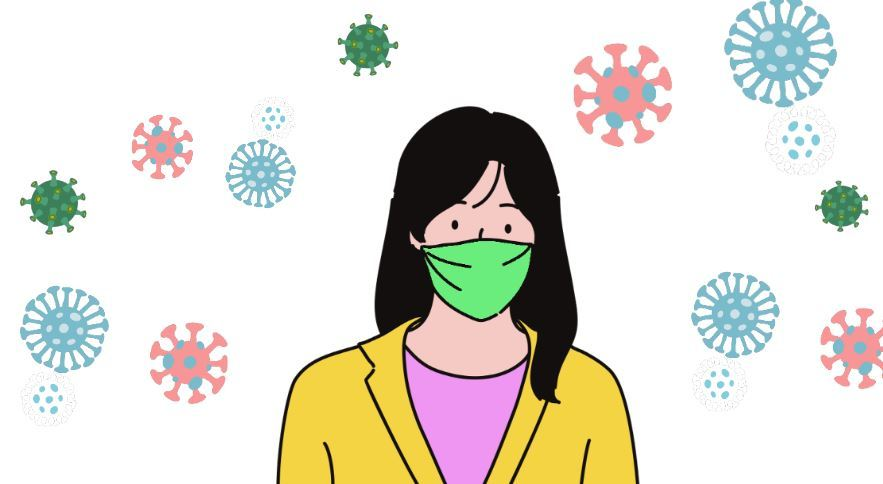# COVID-19 SIR Modelling for Odisha, India

June 12, 2020

By  Abhishek Agrawal

###### Move quickly from one page to another within COVID-19 Series from MATLAB Helper:
Now we are going to show the pandemic analysis of another state of India that is Odisha.As we have discussed in the other SIR Modelling posts, SIR model has only a few tunable parameters. For this case we have selected these ones:

N =10000
????=?
????=?

Wait! What happens with ???? and  ? Sorry, we don't know what their values are. So?

So, let's compute them! This approach includes an algorithm for doing calculations for ???? and ????. We will only need the real data for Odisha.

## Estimating ???? and ???? :

For this, code has been created as shown below.  First, we have to preprocess data
%%%%%%%%%%%%%%%%%%%%%%%%%%%%%%%%%%%%%
% Author: Abhishek Agrawal
% Topic : SIR Modeling using MATLAB
% Company: MATLAB Helper
% Website: https://MATLABHelper.com
% Date : 31-05-2020
% With the help of this script we will plot the actual and the predicted data on COVID-19 cases in ODISHA OR.
%%%%%%%%%%%%%%%%%%%%%%%%%%%%%%%%%%%%%
%% Clearing the command window and workspace and close all other tabs
clc
close
clear
%% Importing the data i.e. COVID-19 cases
url='http://api.covid19india.org/csv/latest/state_wise_daily.csv';
j=1;
i=1;
k=2;
m=3;
% Initial confirmed and recovered cases
% creating the cumilative confirmed and recovered cases arrays for Odisha OR
confirmed (j) =0;
recovered (j) =0;
while (i<=length(raw.OR)-1)
confirmed (j+1) =confirmed(j)+raw.OR(i);
recovered (j+1) =recovered(j)+raw.OR(k)+ raw.OR(m);
j=j+1;
i=i+3;
k=k+3;
m=m+3;
end
%% SIR parameters i.e. Betta and Gamma Calculation
% Declaring beta and gamma as global variable
global beta;
global gamma;
% Total exposed population in ODISHA
N=10000;
infected=confirmed(2:end)' ;
recovered=recovered(2:end)';
susceptible=N-infected-recovered;
% Taking the day as 1 i.e. step size for calculation on daily basis
dt=1;

What does this part of the code mean? Well, First, we use webread for collecting the data. It contains information about susceptible, recovered and infected people for all states of India. This information will be stored in raw variable. So, we need data of Odisha, for getting the data, we use raw.OR (where OR refers to Orissa now known as Odisha). Then we set the population (N), and then we define the variables Beta and Gamma, which will be computed.

Then, a while loop is used to separate the data in infected, recovered, and finally susceptible people. Once it is done. Finally beta and Gamma are computed like as shown with the code below:

% Calulation of parameter Beta
for i=1:(length(susceptible)-1)
beta(i) =(susceptible(i)-susceptible(i+1))/(susceptible(i).*infected(i)*dt ) ;
end
beta(isnan(beta))=0;
beta(isinf(beta))=0;
a=1;
b=1;
gama(b)=0;
betta(a)=0;
for i=1:(length(susceptible)-1)
if(beta(i)~=0)
betta(a+1)=beta(i);
a=a+1;
end
end
beta=betta(end);
% Calculation of parameter Gamma
for i=1:(length(susceptible)-1)
gamma(i) =(recovered(i+1)-recovered(i))/( infected(i)*dt ) ;
end
gamma(isnan(gamma))=0;
gamma(isinf(gamma))=0;
for i=1:(length(susceptible)-1)
if(gamma(i)~=0)
gama(b+1)=gamma(i);
b=b+1;
end
end
gamma=mean(gama);
for l=1:length(susceptible)
if(susceptible(l)~=N)
break
end
end

## Building and Plotting SIR model :

Now we know all the model’s parameters, we are building and plotting the SIR model. Let's consider these functions:

%% Defining the function f
function result = f(u,t)
global beta;
global gamma;
S = u(1); I = u(2); R = u(3);
result = [-beta*S*I beta*S*I-gamma*I gamma*I];
end

The 'result' function is built to represent the SIR model, which is to say, the three differential equations. u variable contains the information about Susceptibles (u(1)), Infected (u(2),) and Recovered (u(3)). Finally, we have the equations which will be needed for the output of the model.

%% Defining the function to solve the ODE
function [u, t] = ode_FE(f, U_0, dt, D)
u = zeros(D+1, length(U_0));
t = linspace(0, D*dt, length(u));
u(1,:) = U_0;      % Initial values
t(1) = 0;
for n = 1:D
u(n+1,:)  = u(n,:) + dt*f(u(n,:), t(n));
end
end

Now,ode_FEfunction computes the next-step values for the Susceptibles, infected and recovered individuals. For this, we need the model (f), initial conditions (U_0), simulation step (dt) which represents days, and finally the total amount of simulation steps (D) which in this case has been defined as 365 days.

Now, let us plot the results. First, we define dt and D as explained previously. Next, the initial values are defined, and using ode_FE we compute SIR values!

%% SIR model to predict the future data
% showing the Simulation for D days
D = 365;
% initial values for susceptible, infected and recovered cases
U_0 = [susceptible(l) infected(l) recovered(l)];
% Differential equations for calculation of future cases
f_handle = @f;
[u, t] = ode_FE(f_handle, U_0, dt, D);
S = u(:,1);
I = u(:,2);
R = u(:,3);
%% Ploting the predicted data
plot(t, S, 'b-', t, I, 'y-', t, R, 'g-')
xlabel('DAYS')
ylabel('Population')
hold on
%% Ploting the actual data
% Inorder to make the length of actual and prdicted data equal assigned other values as NaN
susceptible(end+1:D+1) = nan;
infected(end+1:D+1) = nan;
recovered(end+1:D+1) = nan;
plot(t,susceptible,'m-',t,infected,'r-',t,recovered,'k-');
legend('Predicted-S', 'Predicted-I', 'Predicted-R','Actual-S', 'Actual-I', 'Actual-R');

Finally, real data is plotted and compared with the predicted data of the SIR model.

## Results :Now in the next blog, we will be doing analysis for a different nation, Colombia.

# Conclusion

We have used data untill 31st May 2020 for all these analysis. We have seen some approaches to modelling diseases such as COVID-19 through MATLAB. We have found essential characteristics within this process. We have done Data Analysis and Data Extraction and included a reliable source for the COVID-19 data. We have used preprocessing to extract a subset of the collected data and organise the data accurately. We have done Data Modelling with model construction, i.e. finding the best model that fits the COVID-19 pandemic. We have done the calculation of model parameters using real collected data and finally done the Data Visualisation. All these steps were essential, and luckily, MATLAB has so many functions and tool to use in the given scenario.

Now summing our analysis and coming to a definite conclusion, India's COVID-19 case tally climbed from 100 to one lakh in just 64 days as per the data sourced from the Union Ministry of Health & Family Welfare (MoHFW). On comparing this with global data, sourced from Worldometers, it has been found out that India's COVID-19 growth rate is more than double the number of days as compared to Colombia.

### Important Note

Our COVID-19 codes and analysis have been made for educational and research purpose. We have shown different approaches of Pandemic Modelling for each state and the accuracy of result is not guaranteed for real-life situation. We wish for early end of this pandemic. Now it's in our hands; we need to take our responsibilities & take proper precautions.

Now let's have a quick view of the precautions.

#### Stay Home for non-essential activities!Avoid going to crowded areas. Work From Home and Be A Super HeroMaintain distance while conversing with someone. Avoid touching your face frequentlyWash your hand for 20 seconds.  KEEP A SANTISER ALWAYS with you, wherever you go.While sneezing or coughing, always cover your mouth with a handkerchief or with your arm.

#### Home QuarantineIf you have been facing the symptoms of Cold and Cough or the one's shown with COVID-19, then self-isolate yourself with home quarantine for 14 days.  So stay home stay safe; this is the best way you can contribute to the betterment of the nation.

#### Abhishek Agrawal

I am a tech enthusiast, currently pursuing my Bachelor's under Electronics and Telecommunications Engineering. I have a keen interest in the field of MATLAB, Robotics, IoT, and Embedded systems. Currently working as a MATLAB Developer with MATLAB Helper.

•Nikita Mahoviya says:

Thoroughly explained, this is yet again a masterpiece in the COVID-19 analysis series by MATLAB Helper. Came across so many new functions in all the approaches.

•Aisik De says:

Wonderful blog with proper explanation.

#### Connect with MATLAB Helper ®

Use Website Chat or WhatsApp at +91-8104622179

Watch our Latest Video

Join a Training Module